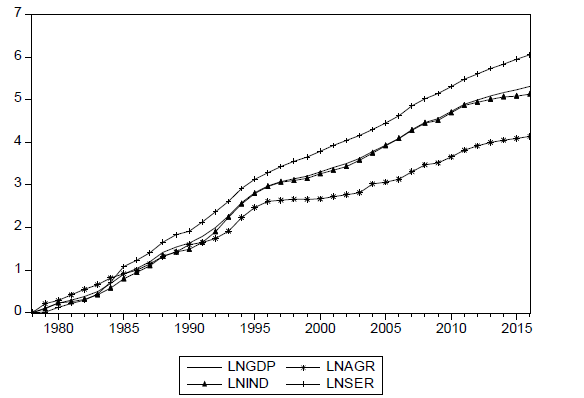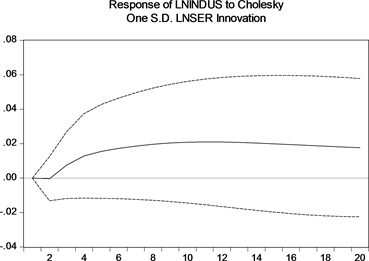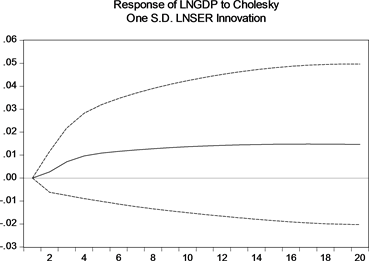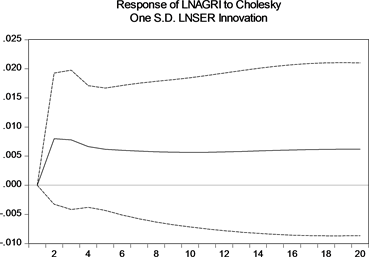﻿ 中国服务业发展与经济增长、农业发展与工业发展关系的实证研究

# 中国服务业发展与经济增长、农业发展与工业发展关系的实证研究Empirical Research of the Relations among Economy Growth, Industrial and Agricultural Development and Service Industry Development

Abstract: The relationship between service industry development and economy growth has been a heated academic topic among scholars. Based on times series data from 1976 to 2016, this paper applies Johansen Co-integration Test, Granger Causality Test Vector Auto-Regressive Model and Impulse Response Function to analyze the relationship among economy growth, industrial and agricultural development, and service industry development. The result shows, 1) service development level has the most significant impact on promoting the economy development; 2) service industry de-velopment has a certain impact on promoting industrial and agricultural development; 3) it takes long time to realize the improvement of GDP from promotion in service industry.

1. 研究背景

2. 实证分析

(一) 数据选取

(二) 单位根检验Figure1. Time series trends of variables (in LN term)

(三) Johanson协整检验

(四) Granger因果检验

Granger因果检验是用于分析时间序列变量之间的因果关系的检验方法。假定两组时间序列变量为 $\left[{x}_{t}\right]$$\left[{y}_{t}\right]$ ，且有关 $\left[{x}_{t}\right]$$\left[{y}_{t}\right]$ 每一变量的预测信息全部包含在这些变量的时间序列当中。构造以下回归方程：

${y}_{t}=\underset{i=1}{\overset{p}{\sum }}{\alpha }_{i}{x}_{i-1}+\underset{j=1}{\overset{q}{\sum }}{\beta }_{i}{y}_{i-1}+{\mu }_{1t}$

${x}_{t}=\underset{i=1}{\overset{s}{\sum }}{\lambda }_{i}{x}_{i-1}+\underset{j=1}{\overset{r}{\sum }}{\gamma }_{i}{y}_{i-1}+{\mu }_{2t}$Table 3. Cointegration test

(五) 向量自回归(VAR)模型

${y}_{t}={\left[\Delta LN{\left(gdp\right)}_{t},\text{\hspace{0.17em}}\Delta LN{\left(\mathrm{arg}\right)}_{t},\text{\hspace{0.17em}}\Delta LN{\left(ind\right)}_{t},\text{\hspace{0.17em}}\Delta LN{\left(ser\right)}_{t}\right]}^{\prime }$

$\text{VAR}\left(2\right)$ 模型的数学表达形式为

${y}_{t}={A}_{1}{y}_{t-1}+{A}_{2}{y}_{t-2}+{\epsilon }_{t}.$Table 4. Granger causality testTable 5. SC and AIC information criterion

(六) 脉冲响应函数Table 6. Estimation results of VAR(2) modelFigure 2. The impulse response of service industry

3. 结论分析

4. 政策建议

(一) 完善法律环境，强化政策支持

(二) 建立统一化的服务标准

(三) 逐步推动服务业市场化进程

(四) 加快行业协会和行业监管机构的建立步伐

 Kuznets, S. (1971) Modern Economic Growth of Nations: Total Outputs and Population Structure. The MIT Press.

 Chenery, H.B. and Syrquin, M. (1975) Patterns of Development, 1950-1970. Oxford University Press.

 郭文杰. 服务业增长, 城市化与经济发展——改革开放后中国数据的经验研究[J]. 当代经济科学, 2006(9).

 李金昌, 程开明. 中国城市化与经济增长的动态计量分析[J]. 财经研究, 2006( 9).

 赵儒煜. 关于产业结构理论问题的思考[J]. 税务与经济, 2003(6).

 刘成林. 现代服务业发展的理论与系统研究[D]: [博士学位论文]. 天津: 天津大学, 2007(12).

 刘丹鹭. 中国服务业生产率及其影响因素研究[D]: [博士学位论文]. 南京: 南京大学, 2012(5).

 杨玉霞. 论中国产业结构的优化[J]. 社会科学辑刊, 2004(1).

 岳希明, 张曙光. 我国服务业增加值的核算问题[J]. 经济研究, 2002(12).

 曾国平, 袁孝科. 中国城市化水平, 服务业发展与经济增长关系实证研究[J]. 财经问题研究, 2010(8).

Top